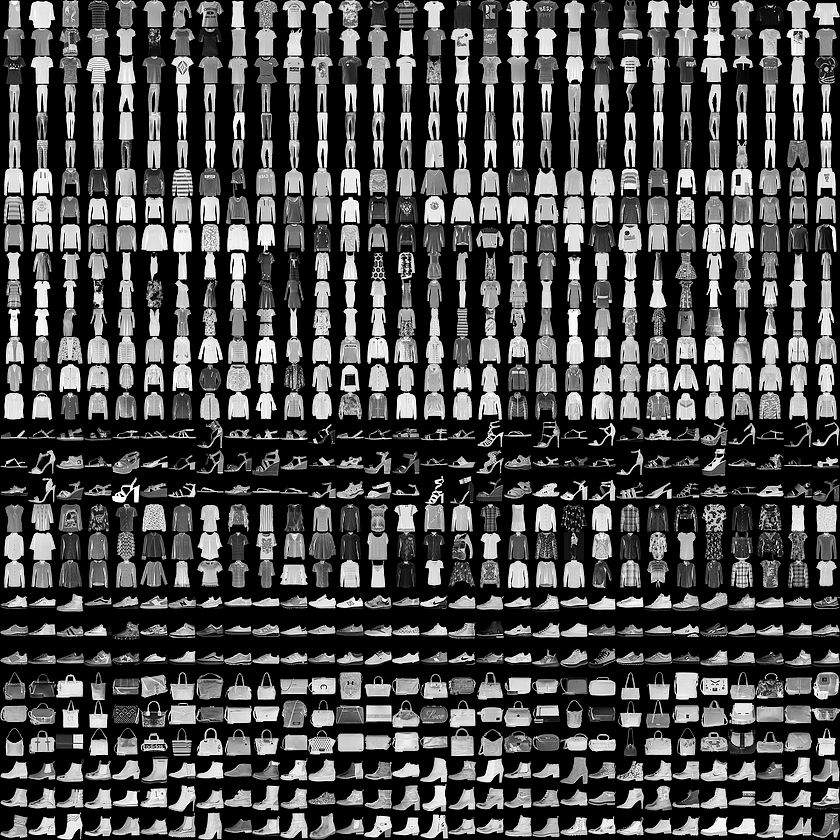# Rumia's blog

Tensorflow官方出了新的文档，经典的MNIST手写数字识别被换成了衣物识别，还挺有意思的，大概整理记录一下

# 训练你的第一个神经网络

## 1.导入相关库

``````from __future__ import absolute_import, division, print_function, unicode_literals

# 导入TensorFlow和tf.keras
import tensorflow as tf
from tensorflow import keras

# 导入辅助库
import numpy as np
import matplotlib.pyplot as plt

``````

tips:

``````from __future__ import ...
# 意思是把下一个版本的特性导入当前版本
``````

## 2.导入 Fashion MNIST数据集Fashion MNIST 旨在替代传统的MNIST数据集 — 它经常被作为机器学习在计算机视觉方向的”Hello, World”。MNIST数据集包含手写数字（0,1,2等）的图像，其格式与我们在此处使用的服装相同。

``````fashion_mnist = keras.datasets.fashion_mnist

(train_images, train_labels), (test_images, test_labels) = fashion_mnist.load_data()  ## 此处可能需要一些小tricks才能正常下载
``````

​ 加载数据集并返回四个NumPy数组:

• `train_images``train_labels`数组是训练集—这是模型用来学习的数据。
• 模型通过测试集进行测试, 即`test_images``test_labels`两个数组。

0 T-shirt/top
1 Trouser
2 Pullover
3 Dress
4 Coat
5 Sandal
6 Shirt
7 Sneaker
8 Bag
9 Ankle boot

``````class_names = ['T-shirt/top', 'Trouser', 'Pullover', 'Dress', 'Coat',               'Sandal', 'Shirt', 'Sneaker', 'Bag', 'Ankle boot']
``````

## 3.探索数据

``````train_images.shape
``````
``````(60000, 28, 28)
``````

``````len(train_labels)
``````
``````60000
``````

``````train_labels
``````
``````array([9, 0, 0, ..., 3, 0, 5], dtype=uint8)
``````

``````test_images.shape
``````
``````(10000, 28, 28)
``````

``````len(test_labels)
``````
``````10000
``````

## 4.数据预处理

``````plt.figure()
plt.imshow(train_images)
plt.colorbar()
plt.grid(False)
plt.show()
````````````train_images = train_images / 255.0test_images = test_images / 255.0
``````

``````plt.figure(figsize=(10,10))
for i in range(25):
plt.subplot(5,5,i+1)
plt.xticks([])
plt.yticks([])
plt.grid(False)
plt.imshow(train_images[i], cmap=plt.cm.binary)   		     plt.xlabel(class_names[train_labels[i]])
plt.show()
``````## 5.构建模型

``````model = keras.Sequential([    keras.layers.Flatten(input_shape=(28, 28)),    keras.layers.Dense(128, activation=tf.nn.relu),    keras.layers.Dense(10, activation=tf.nn.softmax)])
``````

• 损失函数 —这可以衡量模型在培训过程中的准确程度。 我们希望将此函数最小化以”驱使”模型朝正确的方向拟合。
• 优化器 —这就是模型根据它看到的数据及其损失函数进行更新的方式。
• 评价方式 —用于监控训练和测试步骤。以下示例使用准确率(accuracy)，即正确分类的图像的分数。
``````model.compile(optimizer='adam',        loss='sparse_categorical_crossentropy',              metrics=['accuracy'])
``````

1. 将训练数据提供给模型 - 在本案例中，他们是`train_images``train_labels`数组。
2. 模型学习如何将图像与其标签关联
3. 我们使用模型对测试集进行预测, 在本案例中为`test_images`数组。我们验证预测结果是否匹配`test_labels`数组中保存的标签。

``````model.fit(train_images, train_labels, epochs=5)
``````

``````predictions = model.predict(test_images)
``````

``````predictions
``````
``````array([6.6858855e-05, 2.5964803e-07, 5.3627105e-06, 4.5019146e-06,
2.7420206e-06, 4.7881842e-02, 2.3233067e-04, 5.4705784e-02,
8.5581087e-05, 8.9701480e-01], dtype=float32)
``````

``````np.argmax(predictions)
``````
``````9
``````

``````test_labels
``````
``````9
``````

``````def plot_image(i, predictions_array, true_label, img):  predictions_array, true_label, img = predictions_array[i], true_label[i], img[i]  plt.grid(False)  plt.xticks([])  plt.yticks([])    plt.imshow(img, cmap=plt.cm.binary)    predicted_label = np.argmax(predictions_array)  if predicted_label == true_label:    color = 'blue'  else:    color = 'red'    plt.xlabel("{} {:2.0f}% ({})".format(class_names[predicted_label],                                100*np.max(predictions_array),                                class_names[true_label]),                                color=color)def plot_value_array(i, predictions_array, true_label):  predictions_array, true_label = predictions_array[i], true_label[i]  plt.grid(False)  plt.xticks([])  plt.yticks([])  thisplot = plt.bar(range(10), predictions_array, color="#777777")  plt.ylim([0, 1])  predicted_label = np.argmax(predictions_array)    thisplot[predicted_label].set_color('red')  thisplot[true_label].set_color('blue')
``````

``````i = 0plt.figure(figsize=(6,3))plt.subplot(1,2,1)plot_image(i, predictions, test_labels, test_images)plt.subplot(1,2,2)plot_value_array(i, predictions,  test_labels)plt.show()
````````````i = 12plt.figure(figsize=(6,3))plt.subplot(1,2,1)plot_image(i, predictions, test_labels, test_images)plt.subplot(1,2,2)plot_value_array(i, predictions,  test_labels)plt.show()
````````````# 绘制前X个测试图像，预测标签和真实标签# 以蓝色显示正确的预测，红色显示不正确的预测num_rows = 5num_cols = 3num_images = num_rows*num_colsplt.figure(figsize=(2*2*num_cols, 2*num_rows))for i in range(num_images):  plt.subplot(num_rows, 2*num_cols, 2*i+1)  plot_image(i, predictions, test_labels, test_images)  plt.subplot(num_rows, 2*num_cols, 2*i+2)  plot_value_array(i, predictions, test_labels)plt.show()
````````````# 从测试数据集中获取图像img = test_imagesprint(img.shape)
``````
``````(28, 28)
``````

`tf.keras`模型经过优化，可以一次性对批量,或者一个集合的数据进行预测。因此，即使我们使用单个图像，我们也需要将其添加到列表中:

``````# 将图像添加到批次中，即使它是唯一的成员。img = (np.expand_dims(img,0))print(img.shape)
``````
``````(1, 28, 28)
``````

``````predictions_single = model.predict(img)print(predictions_single)
``````
``````[[6.6858927e-05 2.5964729e-07 5.3627055e-06 4.5019060e-06 2.7420206e-06
4.7881793e-02 2.3233047e-04 5.4705758e-02 8.5581087e-05 8.9701480e-01]]
``````
``````plot_value_array(0, predictions_single, test_labels)plt.xticks(range(10), class_names, rotation=45)plt.show()
```````model.predict`返回一个包含列表的列表，每个图像对应一个列表的数据。获取批次中我们(仅有的)图像的预测:

``````prediction_result = np.argmax(predictions_single)print(prediction_result)
``````
``````9
``````